﻿ Offset Method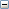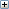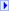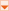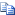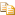Collapse All Expand All
dx
Required. A Double that represents the distance to move the rectangle horizontally. Negative values move the rectangle to the left.
dy
Required. A Double that represents the distance to move the rectangle vertically. Negative values move the rectangle down.
 Rectangle Object : Offset Method

Glossary Item Box

#Description

Moves the rectangle by the specified amount horizontally and vertically, in units of the coordinate system of the current map.

#Syntax

`object.Offset ( dX, dY )`

#Parameters

dx
Required. A Double that represents the distance to move the rectangle horizontally. Negative values move the rectangle to the left.
dy
Required. A Double that represents the distance to move the rectangle vertically. Negative values move the rectangle down.

#Example

Pans the map by moving its extent.
Offset Example (VBScript)Copy Code
```Sub ShiftMap
Dim objRect, dblDeltaX, dblDeltaY
'Set the rectangle to the current map extent
Set objRect = Application.Map.Extent
'Calculate the offset relative the current map extent
dblDeltaX = objRect.Width * 0.1
dblDeltaY = objRect.Height * 0.1
'Offset the rectangle
Call objRect.Offset (dblDeltaX, dblDeltaY)
'Re-set the map extent to the rectangle
Application.Map.Extent = objRect
Set objRect = Nothing
End Sub```